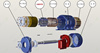PMSM（Permanent Magnetic Synchronous Machine） 

• 一、PMSM的基本的构造PMSM（permanent magnet synchronous motor）实际工作是一种交流电机，其定子运行是三项的相差的交流电，而转子则是永磁体。但是这种电机最大的优势就是交流电能量由直流提供，这样就可以对电机...
一、PMSM的基本的构造
PMSM（permanent magnet synchronous motor）实际工作是一种交流电机，其定子运行是三项的相差 的交流电，而转子则是永磁体。但是这种电机最大的优势就是交流电能量由直流提供，这样就可以对电机进行精确的控制，而且解决了电刷带来的寿命问题。
下面对其工作原理进行简单的介绍，如图 1，定子的工作电流都为正弦波，而且其三项在任何时候相加都为零，所以PMSM中三项绕组实际上没有中线的，其在电机中示例绕线方法如图 2，所以实际上在PMSM中XYZ是连接在一个点的。图 1 PMSM转子电流

图 2 PMSM基本的绕线方法

从绕线的图 2中不难看出，实际的电流方向产生的磁场是和转子磁场在同一个平面，这也就是PMSM控制的基本需要和基本方法。从图中也不难看出，实际在A相产生的磁场在开始是需要与转子磁极的D轴方向相反（可以相差一个确定的角度，软件实现），确切的说应该是必须知道转子的D轴的位置。这个问题实际在控制中是开始的定向问题，在这里简单的介绍一下方法：如果位置传感器是绝对码盘或者旋变，则可根据绝对位置处理，如果是增量码盘，则需要开始的一个UVW的大概位置估算。
除此之外，这里还需要明白几个原理性的问题，这里啰嗦一下：很多人从事这一块的研发在知道怎么处理整个系统的过程而实际上是对整个基础原理模糊的，这也就是很多国人做研发的通病，只知道怎么做，从来不知道为什么这么做以致永远只是模仿而不可能创新或者改进。言归正传，首先我们知道在控制过程中需要检测电流，然后进行clarke和park变换，从而出现了电流方向问题，人家这么说是为了方便，而实际上上这里的电流方向不是电流方向，而是电流产生的电磁场方向（这是因为电磁场的大小与产生它的电流方向成正比的）。然后研究一下电压的概念，绕组电压是比电流相位超前 的，而很多我们需要的结果是与电压成一定简单关系的，这是因为电压是场量，而电流不是。根本上没有电压这个东西，它只是间接反应电流的一种我们定义出来的表达方式，所以它的变化影响电流，而电流的变化会在场的方面反应在电压上。比如前面讨论的问题，在电机初始的时候，A相电流是零，这是因为在绕组上电流不能突变，而这个时候电压是最大，反应了实际我们是需要加在绕组上最大的我们需要的一个量（电流）。这里描述的有些晦涩，但是物理这个自然界基本规律实际就是这么反应的，希望读我文章的读者能自己仔细揣摩，反应一个自然界的问题（都上升到哲学问题了）。
二、PMSM的基本工作原理
如果你明白了我前面讨论的PMSM的基本问题，这里的东西实际相对就很简单，它的工作原理实际就是用直流电机的方法进行控制交流电机。
1)        首先我们看看这里涉及的第一个问题，定向问题。永磁同步电机的是通过转子定向工作的，也就是说我们控制的所有参考点是以转子为参考的。
所以我们开始控制之前就必须先知道我们参考点的位置，实际中大多工程是通过位置传感器来获得转子位置的，前面也已经提到除了旋变一般采用码盘作为位置传感器，而码盘又有绝对码盘和增量码盘（价格决定），而这两种的初始位置处理方法也不尽相同，但是都很简单。
2)        第二个问题就是它通过IGBT将直流电源转换为电机需要的三项交流电的，所以IGBT也就是这里决定电机控制好坏的一个关键器件，但是IGBT的开关时间实际是不一样的，所以会产生上下管子导通而短路的问题，实际工程中通过加死区来解决这个问题的，但是死区却给三项交流电的形成带来了不光滑的问题，这也是PMSM一个一直未得到很好解决的问题。
我以前做过一个十二矢量的控制方法，从理论上可以完全消除死区问题，而且会提高正弦波的精度。但是因为IGBT的反向二极管保护问题产生畸变，努力以后能改变IGBT的工作原理而实现这个突破性的问题，也是在工控行业的一个期待。
3)        下面讨论一下具体PMSM的控制方法，它是通过六矢量的空间矢量控制实现的（SVPWM）,先看其控制方程如下：所以可以计算得功率得大小为：由于变换不是等功率变换，所以以上也只是系统一个有功功率的体现，并且只有第三相与转子的运动状态有关且直接参与机电能量转换的电磁功率。故电磁功率表示为：由于我们的伺服系统大多采用最大力矩控制方法，所以令所以可以通过控制 对整个系统进行控制，如下面框图：后面我会对具体这个框图的实现写相关的文章，并且会针对伺服系统中存在的很多问题进行讨论和解决。由于本人知识水平有限，不足之处希望大家指正和共同学习，我的邮箱是：gsujianwen@163.com。如果有问题可以和我联系。
展开全文• 永磁同步电机控制原理 矢量控制框图如下图所示： 矢量控制的原理是在永磁同步电机上设法模拟直流电动机的转矩控制规律，经过坐标变换，使其电流矢量分解为产生磁通的电流分量和产生转矩的电流分量，两个...
注：
1：此为永磁同步控制系列文章之一，应大家的要求，关于永磁同步矢量控制的系列文章已经在主页置顶，大家可以直接去主页里面查阅，希望能给大家带来帮助，谢谢。
2：矢量控制的六篇文章后。弱磁、MTPA、位置控制系列讲解已经补充，也放在主页了，请大家查阅。
3: 恰饭一下，也做了一套较为详细教程放在置顶了，内含基本双闭环、MTPA、弱磁、三闭环、模糊PI等基本控制优化策略，也将滑模，MRAS等无速度控制课题整理完成，请大家查看_
2  永磁同步电机控制原理
2.1 从PMSM电机的数学模型出发。
dq 轴 电压方程：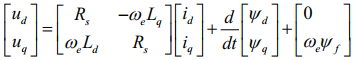dq 轴 轴磁链方程：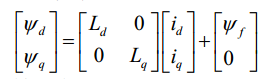dq 轴 转矩方程：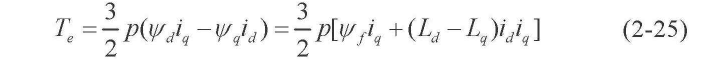dq 轴 运动方程：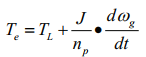分析上述方程，如果我们能够控制 id=0
那么电压方程就可简化为：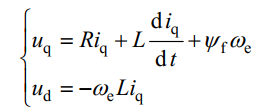转矩方程为：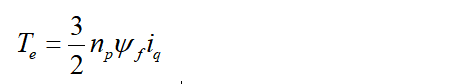运动方程为：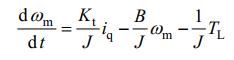以上式中：ψf 是永磁体磁链，R 和 L 是定子绕组的电阻电感，we 是电机电角速度
，wm 是电机的机械角速度，p 为极对数，kt 是转矩常数，J 为转动惯量，B 为摩擦系数，Tl 是负载系数。
从以上方程可以看出，仅控制 iq 我们就可以控制转矩的大小，d轴电压也仅与 iq有关，这样极有益于我们的控制。
并且，当 id=0 时，相当于一台典型的他励直流电动机，定子只有交轴分量，且定子磁动势的空间矢量正好和永磁体磁场空间矢量正交。所以为了减少损耗，完全可以将id=0，降低铜耗。
矢量控制框图如下图所示：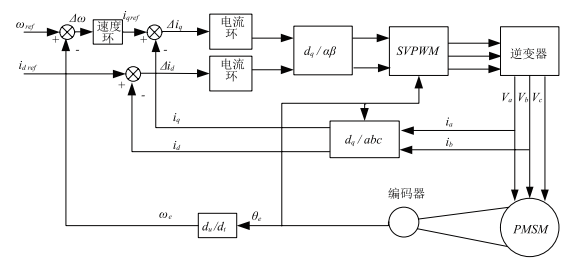小结：
矢量控制的原理是在永磁同步电机上设法模拟直流电动机的转矩控制规律，经过坐标变换，使其电流矢量分解为产生磁通的电流分量和产生转矩的电流分量，两个分量互相垂直，相互独立。这样就可以对它们进行单独调节，与直流电动机的双闭环控制系统类似。（双闭环控制系统在陈伯时电力拖动控制书2.4章节有详细的介绍，大家可以回顾一下。）
2.2 坐标变换*（参考于陈伯时电力拖动自动控制系统6.6.3章节）*
2.2.1 进行坐标变换的原因

永磁同步电机中，定子磁势Fs、转子磁势Fr、气隙磁势之间的夹角都不是90°，耦合性强，根本无法对磁场和电磁转矩进行独立控制
直流电机励磁磁场垂直于电枢磁势，二者各自独立，互不影响
直流电机控制策略多种多样，能够使其应对不同场合

所以将永磁同步电机的数学模型分析后，进行坐标变换将其模拟为直流电机进行控制，会很大程度上提高电机可控性和运行效率。
2.2.2 坐标变换基本思路**
*

不同电机模型等效的原则：在不同坐标系所产生的磁动势完全一致。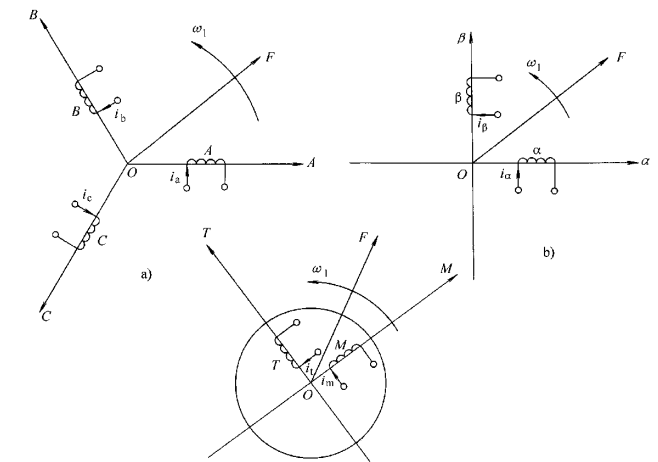如上图中a中，电机通入三相平衡的正弦电流时，所产生的合成磁动势是旋转磁动势，它在空间上是呈正弦分布的，以同步转速w1顺着A-B-C的顺序进行旋转。而旋转磁动势，并不是只有三相绕组才可以产生，通入平衡的多相电流都可以产生想要的旋转电磁场，其中两相的最为简便。只需要通入时间上互查90°的平衡交流电就可以产生旋转磁场。如果控制a中和b中的旋转磁动势的大小和转速都相同，那么即可认为二者等效。
在看c图，两个相互垂直的绕组M和T，其中通以电流im和it，产生合成磁动势F，显然这个磁动势相对于M和T绕组是固定的，这个时候如果人为的将两个绕组在内的整个铁芯按照以上同步转速旋转，那么即可以产生跟三相绕组等效的旋转磁场。如果假设有人站在这个铁芯上看，这个电机的模型就完全与直流电机等效了。
磁动势的等效也就代表着电流的等效，iA/iB/iB 、ia/ib 和 im/it等效，他们三者能产生相同的磁动势，现在最重要的任务就是找到 以上三组电流之间准确的等效关系。
2.3 3相静止-两相静止变换——3/2变换

物理基础：各相磁动势=有效匝数 * 电流大小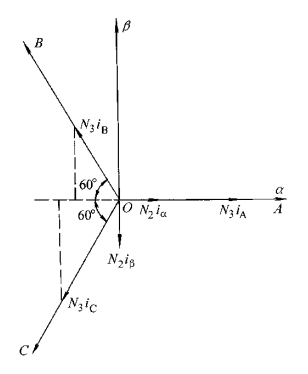如上图所示，为方便起见，将A相与a相重合，ABC为三相静止磁动势矢量图，ab为两相静止磁动势矢量图。
当两者磁动势相等时，两套绕组瞬时磁动势在ab轴上的投影相等。
即有以下关系式：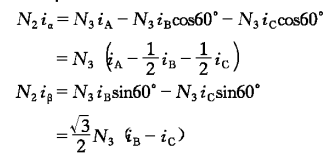由陈伯时书籍附录4所证明，变换前后功率不变时，三相和两相的匝数比为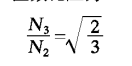结合以上二式可得变换矩阵为：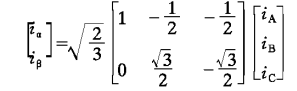若三相绕组是Y形联结不带零线，那么ia+ib+ic=0，代入上式可得变换矩阵：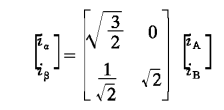2.4 两相静止-两相旋转变换——2s/2r变换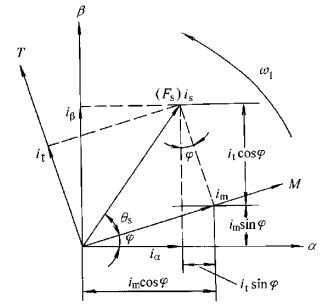如上图所示，ab 为两相静止坐标系，MT 为两相旋转坐标系;
MT坐标系以同步转速 w1 旋转，且 it 和 im 的长度不变（由于匝数相等约去）。
而 ab 坐标系是静止不动的，a轴和M轴之间的夹角ψ随着时间而改变，
由此可推算，要使二者磁动势相等效，it 和 im 在 a 轴和 b 轴上的投影要与 ia 和 ib 等效，即可得出：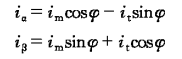从而可得出 两相旋转变两相静止的变换矩阵为：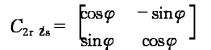通过对矩阵变换，或者更换公式两边的位置，可得两相静止变两相旋转坐标系为：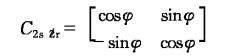小结：
永磁同步电机系统是一个非线性系统，通过数学变换，将这个系统拟化成一个他励直流电机模型来控制，会很大程度上降低控制难度，这是控制策略的核心。
而坐标变换的核心是不同坐标系产生磁动势一致；通过各个坐标系之间的等量关系，计算出我们需要的变换矩阵。
有了坐标变换，有了拟化的他励直流电机模型，我们下一步就是进行电流环和转速环的设计了。
后续文章链接：
永磁同步电机矢量控制到无速度传感器控制学习教程（PMSM）
永磁同步电机矢量控制（一）——数学模型
永磁同步电机矢量控制（三）——电流环转速环 PI 参数整定
永磁同步电机矢量控制（五）——波形记录及其分析
永磁同步电机矢量控制（六）——MTPA最大转矩电流比控制
永磁同步电机矢量控制（七）——基于id=0的矢量控制的动态解耦策略
永磁同步电机矢量控制（八）——弱磁控制(超前角弱磁)
永磁同步电机矢量控制（九）——三闭环位置控制系统
永磁同步电机矢量控制（十）——PMSM最优效率（最小损耗）控制策略


展开全文坐标变换 控制原理
• 一、坐标变换 1.1. 三相静止坐标系(abcabcabc)和两相静止坐标系(α/β\alpha/\betaα/β)之间的变换 根据图中所示abcabcabc坐标系和αβ\alpha\betaαβ坐标系之间的关系，可以列出以下等式 ...
最近开始学习电机控制，仅以此记录自己的学习进度。
一、坐标变换
1.1. 三相静止坐标系($abc$)和两相静止坐标系($\alpha/\beta$)之间的变换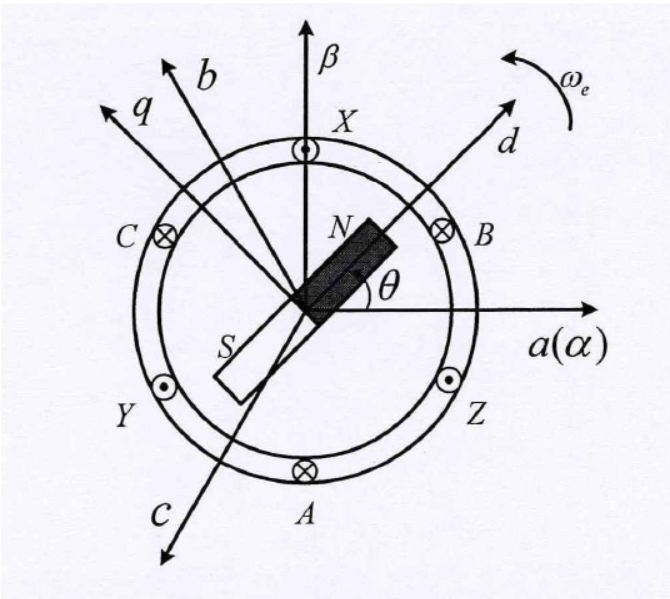根据图中所示$abc$坐标系和$\alpha\beta$坐标系之间的关系，可以列出以下等式
$U_\alpha = U_a - U_bcos(\frac \pi3) - U_ccos(\frac \pi3) \\ U_\beta = U_bcos(\frac \pi6) - U_ccos(\frac \pi6)$
化简即可得到$abc$坐标系转为$\alpha\beta$坐标系的变换矩阵，即$Clarke$变换：
$\left[ \begin{matrix} U_\alpha\\ U_\beta\\ \end{matrix} \right]= K\left[ \begin{matrix} 1 & -\frac12 & -\frac12 \\ 0 & \frac {\sqrt3}2 & -\frac {\sqrt3}2 \\ \end{matrix} \right] \left[ \begin{matrix} U_a\\ U_b\\ U_c\\ \end{matrix} \right] \tag {1.1}$
考虑变换前后的幅值相等，则式中$K$等于$\frac 23$；如果要求变换前后功率等，则式中$K$等于$\sqrt\frac 23$。很多资料都没有详细说明这两种变换的系数是怎么来的，一度让我很疑惑。通过查资料和推导，终于怎么回事了。恒幅值变换是指$U_\alpha$的幅值和$U_a$相等，而恒功率变换是指变换前后的功率相等，下面给出推导过程。
设三相电压是平衡的，其幅值为$U_{max}$，则：
$\left[ \begin{matrix} U_a \\ U_b \\ U_c \end{matrix} \right]= U_{max}\left[ \begin{matrix} cos(\varphi) \\ cos(\varphi - \frac {2\pi}3) \\ cos(\varphi + \frac {2\pi}3) \end{matrix} \right] \tag {1.2}$
将式$(1.2)$代入式$(1.1)$中，可以得到$U_\alpha$的表达式如下：
$U_\alpha = U_{max}(cos\varphi - \frac 12cos(\varphi - \frac {2\pi}3)- \frac 12cos(\varphi + \frac {2\pi}3) = \frac 32cos\varphi$
可见变换后的$U_\alpha$幅值是变换前$U_a$的1.5倍。因此，为了使$U_a$的幅值与$U_\alpha$的幅值相等，则需要在变换矩阵前乘以$\frac 23$。
设变换前的电压有效值是$U$，电流有效值是$I$,则容易得出变换后的有效值是$1.5U$，电流有效值是$1.5I$。可以分别得出变换前后的功率$P_1$和$P_2$：
$P_1 = U*I*3 = 3UI\\ P_2 = 1.5U*1.5I*2 = 4.5UI$
可见变换前后的功率不相等，需要给变换矩阵乘以一个系数$K$使其相等。当变换矩阵乘以系数$K$之后，变换前后的功率的表达式如下：
$P_1 = U*I*3 = 3UI \\ P_2 = 1.5*K*U*1.5*K*i*2 = 4.5K^2UI$
令式中$P_1 = P_2$,即可得到$K = \sqrt{\frac 23}$。
因此，在式$(1.1)$中，当$K=\frac 23$,则为恒幅值变换；当$K=\sqrt{\frac 23}$,则为恒功率变换。
根据同样的思路，或者可以得到$Clark$反变换表达式：
$\left[ \begin{matrix} U_a\\ U_b\\ U_c\\ \end{matrix} \right]= K\left[ \begin{matrix} 1 & 0\\ -\frac 12 & \frac {\sqrt3}2\\ -\frac 12 & -\frac {\sqrt3}2]\\ \end{matrix} \right] \left[ \begin{matrix} U_\alpha\\ U_\beta \end{matrix} \right] \tag {1.3}$
其中，当$K=\frac 23$,则为恒幅值变换；
当$K=\sqrt{\frac 23}$,则为恒功率变换。
式$(1.1)$中的矩阵和式$(1.3)$中的矩阵相乘的结果是单位矩阵。
1.2 两相静止坐标系($\alpha\beta$)和两相旋转坐标系($dq$)之间的变换
根据图中所示$\alpha\beta$坐标系和$dq$坐标系之间的关系，可以列出以下等式:
$U_d = U_\alpha cos\theta + U_\beta sin\theta\\ U_q = -U_\alpha sin\theta + U_\beta cos\theta$
于是可以得到$\alpha\beta$坐标系转换为$dq$坐标系的变换矩阵，即$Park$变换：
$\left[ \begin{matrix} U_d\\ U_q \end{matrix} \right]= \left[ \begin{matrix} cos\theta & sin\theta \\ -sin\theta & cos\theta \\ \end{matrix} \right] \left[ \begin{matrix} U_\alpha \\ U_\beta \end{matrix} \right] \tag {1.4}$
同理，由图中也可以将$\alpha\beta$坐标系下的向量由$dq$坐标表示：
$U_\alpha = U_dcos\theta - U_qsin\theta \\ U_\beta = U_dsin\theta + U_qcos\theta \\$
于是可以得到$dq$坐标系转换为$\alpha\beta$坐标系的变换矩阵，即$Park$反变换：
$\left[ \begin{matrix} U_\alpha \\ U_\beta \end{matrix} \right]= \left[ \begin{matrix} cos\theta & -sin\theta \\ sin\theta & cos\theta \end{matrix} \right] \left[ \begin{matrix} U_d \\ U_q \end{matrix} \right] \tag{1.5}$
1.3 三相静止坐标系（$abc$）和两相旋转坐标系($dq$)之间的变换
结合$Clarke$变换和$Park$变换可以得到$abc$坐标系和$dq$坐标系之间的变换如下：
$\left[ \begin{matrix} U_d \\ U_q \end{matrix} \right]= K\left[ \begin{matrix} cos\theta & cos(\theta - \frac {2\pi}3) & cos(\theta + \frac {2\pi}3) \\ -sin\theta & -sin(\theta - \frac {2\pi}3) & -sin(\theta + \frac {2\pi}3) \end{matrix} \right] \left[ \begin{matrix} U_a\\ U_b\\ U_c \end{matrix} \right] \tag{1.6}$
恒幅值变换时，$K=\frac 23$;恒功率变换时，$K = \sqrt {\frac 23}$。
其反变换为：
$\left[ \begin{matrix} U_a\\ U_b\\ U_c \end{matrix} \right]= K\left[ \begin{matrix} cos\theta & -sin\theta \\ cos(\theta - \frac {2\pi}3) & -sin(\theta - \frac{2\pi}3) \\ cos(\theta + \frac {2\pi}3) & -sin(\theta + \frac{2\pi}3) \end{matrix} \right] \left[ \begin{matrix} U_d\\ U_q \end{matrix} \right] \tag{1.7}$
恒幅值变换时，$K＝1$;恒功率变换时，$K = \sqrt {\frac 23}$。
二、永磁同步电机的数学模型
2.1 永磁同步电机在三相静止$(abc)$坐标系下的数学模型
永磁同步电机在三相静止坐标系下的磁链方程为
$\left[ \begin{matrix} \psi_a \\ \psi_b \\ \psi_c \end{matrix} \right]= \left[ \begin{matrix} L_{aa} & M_{ab} & M_{ac}\\ M_{ba} & L_{bb} & M_{bc}\\ M_{ca} & M_{cb} & L_{cc}\\ \end{matrix} \right] \left[ \begin{matrix} i_a\\ i_b\\ i_c \end{matrix} \right] + \psi_f\left[ \begin{matrix} cos\theta \\ cos(\theta - \frac {2\pi}3) \\ cos(\theta + \frac {2\pi}3] \end{matrix} \right] \tag {2.1}$
式中:
$\psi_f$——永磁体磁链；
$\theta$——电机转子磁极位置，即永磁体N极与$a$相轴线之间的夹角；
$L_{aa}、L_{bb}、L_{cc}$——定子绕组的自感，且在理想情况下，$L_{aa} = L_{bb} = L_{cc}$;
$\psi_a、\psi_b、\psi_c$——三相静止坐标系下的定子磁链；
$M_{ab}、M_{ac}、M_{ba}、M_{bc}、M_{ca}、M_{cb}$——定子三相绕组间的互感。
永磁同步电机在三相静止坐标系下的定子电压方程为：
$\left[ \begin{matrix} u_a \\ u_b \\ u_c \end{matrix} \right]= \left[ \begin{matrix} R_s & 0 & 0 \\ 0 & R_s & 0 \\ 0 & 0 & R_s \end{matrix} \right] \left[ \begin{matrix} i_a \\ i_b \\ i_c \end{matrix} \right] + p\left[ \begin{matrix} \psi_a \\ \psi_b \\ \psi_c \end{matrix} \right] \tag {2.2}$
式中:
$u_a、u_b、u_c$——定子三相电压；
$R_s$——定子电阻；
$i_a、i_b、i_c$——定子三相电流;
$p$——微分算子，表示对时间的微分。
式$(2.2)$的物理意义表明，定子三相电压是由定子电阻上的电压和电感（包括自感和互感）电压相加得来的。
永磁同步电机在三相静止坐标系下的转矩方程为：
$T_e = \frac 12 p_n \psi_f (i_acos\theta + i_bcos(\theta - \frac {2\pi}3)+i_ccos(\theta + \frac {2\pi}3)) \tag {2.3}$
式中：
$T_e$——电机的电磁转矩；
$p_n$——电机的极对数。
电机的运动方程为：
$T_e = T_L + \frac Jp_n\frac {d\omega_e}{dt} + \frac B{p_n} \omega_e + \frac K{p_n}\theta \tag {2.4}$
式中：
$T_L$——负载转矩；
$B$——摩擦系数；
$K$——扭矩系数；
$J$——转动惯量。
$\omega_e$——电气角速度。与机械角速度的关系是：$\omega_e = p_n\omega$。
2.2 永磁同步电机在两相同步旋转$(dq)$坐标系下的数学模型
永磁同步电机在两相同步旋转坐标系下的电压方程为：
\left\{ \begin{aligned} u_d = R_si_d+p\psi_d-\omega_r\psi_q \\ u_q = R_si_q + p\psi_q +\omega_r\psi_d \end{aligned} \right. \tag{2.5}
式中：
$u_d、u_q、i_d、i_q、\psi_d、\psi_q$——分别表示定子d轴和q轴的电压、电流、磁通；
$p$——为微分算子，表示对时间的微分；
$\omega_r$——转子的电角速度。
推导过程如下：
根据$(1.3)$节中给出的两相旋转坐标系到三相静止坐标系中的变换公式，取A相单独分析，可以得到：
\left\{ \begin{aligned} & u_a = K(u_dcos\theta - u_qsin\theta) \\ & i_a = K(i_dcos\theta - i_qsin\theta) \\ & \psi_a = K(\psi_dcos\theta - \psi_qsin\theta) \end{aligned} \right. \tag{2.6}
由$2.1$节可知，在三相静止坐标系下的A相电压方程为：
$u_a = i_aR_s + p\psi_a \tag{2.7}$
将式$(2.6)$中的表达式代入式$(2.7)$中，整理后可得：
$(u_d - R_si_d-p\psi_d+\psi_qp\theta)cos\theta - (u_q-R_si_q - p \psi_q - \psi_dp\theta)sin\theta = 0 \tag{2.8}$
位置角度$\theta$的微分便是旋转角速度，即：
$p\theta = \omega_r \tag{2.9}$
对于式$(2.8)$来说，由于位置角度$\theta$为任意值，因此下列两个式子分别成立：
\left\{ \begin{aligned} u_d = R_si_d+p\psi_d-\omega_r\psi_q \\ u_q = R_si_q + p\psi_q +\omega_r\psi_d \end{aligned} \right.
推导完毕。
磁链方程为：
\left\{ \begin{aligned} &\psi_d = L_di_d+\psi_f \\ &\psi_q= L_qi_q \end{aligned} \right.
转矩方程为：
$T_e = Kp_n[\psi_fi_q + (L_d-L_q)i_di_q]$
当$K=\frac 32$,则为恒幅值变换；当$K=1$,则为恒功率变换。
在三相坐标系下的复杂的电感耦合关系，在DQ坐标系下不复存在。但$L_d$和$L_q$与三相坐标系下的各种电感关系还没有理清楚。


展开全文坐标变换 数学模型
• 在学习FOC控制前，我对于FOC控制完全不懂，只知道中文叫做磁场定向控制，因公司产品开发需要用到对永磁同步电机(PMSM)进行精确的位置控制，才开始从网上了解什么是FOC，有哪些数学公式，控制的过程是怎么样的，但...
      在学习FOC控制前，我对于FOC控制完全不懂，只知道中文叫做磁场定向控制，因公司产品开发需要用到对永磁同步电机(PMSM)进行精确的位置控制，才开始从网上了解什么是FOC，有哪些数学公式，控制的过程是怎么样的，但由于公司没有人知道这一块的知识，所以只能一个人慢慢找资料学习，网上有不少关于FOC的资料，不过讲的都不全面，而且有的还会存在错误，但是不懂的时候也无法分辨对错，所以走了不少弯路。所以将个人的学习心得记录于此，与大家分享，由于需要对电机进行位置控制，所以使用了14位分辨率的磁编码器。

FOC主要是通过对电机电流的控制实现对电机转矩(电流)、速度、位置的控制。通常是电流作为最内环，速度是中间环，位置作为最外环。

下图是电流环(最内环)的控制框图：

在图一中，Iq_Ref是q轴(交轴)电流设定值，Id_Ref是d轴(直轴)电流设定值，关于交轴直轴不再介绍，大家自行百度。

在得到三相电流和电角度后，即可以进行电流环的执行了：三相电流Ia, Ib, Ic经过Clark变换得到Iα, Iβ；然后经过Park变换得到Iq, Id；然后分别与他们的设定值Iq_Ref, Id_Ref计算误差值；然后分别将q轴电流误差值代入q轴电流PI环计算得到Vq，将d轴电流误差值代入d轴电流PI环计算得到Vd；然后对Vq, Vd进行反Park变换得到Vα, Vβ；然后经过SVPWM算法得到Va, Vb, Vc，最后输入到电机三相上。这样就完成了一次电流环的控制。

当对PMSM进行速度控制时，需要在电流环外面加一个速度环，控制框图如下：

在图二中，Speed_Ref是速度设定值，ω是电机的转速反馈，可以通过电机编码器计算得到。

将计算得到的电机速度ω与速度设定值Speed_Ref进行误差值计算，代入速度PI环，计算的结果作为电流环的输入；比较图二和图一的电流环部分可以发现，图二中d轴电流被设定为零(Id_Ref=0)，因为d轴电流对于驱动电机的转动不会产生输出力，所以通常情况下都会将d轴电流设定为零(但不是总是设定为0的)；当Id_Ref=0时，Iq_Ref就等于了速度环的输出；再结合上面的电流环，就实现了速度电流的双闭环控制。

当对PMSM进行位置控制时，需要在速度电流环外面加一个位置环，控制框图如下：

在图三中，Position_Ref是位置设定值，Position(θ)是电机的当前位置，可以通过电机编码器得知，位置控制可以分为电角度位置控制和机械角度位置控制。

将得到的当前位置Position(θ)和位置设定值Position_Ref计算误差值代入P环，输出作为速度环的输入Speed_Ref,在结合上面的速度电流环实现位置速度电流三闭环控制。

在实际使用中，由于磁编码器无法直接返回电机转速ω，需要计算一定时间内的磁编码值变化量来表示电机的转速ω(M法测速)，假设1ms的角度变化量为δ个，则ω=δ/1ms=δ,(单位：个/ms)，当电机转速比较快的时候，这样的方式是可以的；但是在位置控制的时候，电机的速度会很慢，1分钟的转速可能只有1、2转，用M法测速会存在非常大的误差，增大单位时间可以适当降低误差，但随之而来整个系统的延迟也会增大。

所以为了避免速度环节带来的误差以及系统延迟带来的影响，只使用位置和电流组成的双环进行控制，不过此时需要对位置环做一定的变化，控制框图如下：

在图四中，只使用了位置电流双环实现位置控制。

在位置控制中，会涉及到电机的启动加速和刹车减速，所以只有P环肯定是不够的，还需要加入I和D实现PID环进行控制；如果对于位置控制的精度要求不高，允许存在1、2度的误差的话，可以只使用P和D实现PD环控制即可。

以上简单介绍了电机控制中的过程，不难发现，主要包括了PID控制器和FOC控制算法。PID控制器是自动控制中最常用的一种控制算法，应用非常广泛，网上关于PID的资料也非常多，下面详细讲解FOC控制算法。

从上面的控制框图中可以看出，FOC主要包含了电流采样、坐标变换(Clark, Park, 反Park)、SVPWM。

前面讲过，三相电流Ia, Ib, Ic是可以通过采样和公式Ia+Ib+Ic=0得到的，并三相电流的相位差是120°，如下图：

图五中Ia, Ib, Ic分别是三相电流。

然后经过Clark变换得到Iα, Iβ，如下图：

Clark变换是将静止的三相a, b, c变换成静止的两相α, β，由于不知道如何在编辑器中输入矩阵，所以选择在word中写好截图过来，公式如下：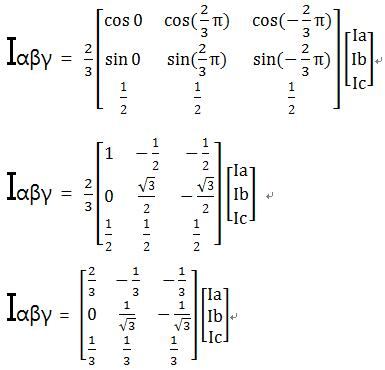于是可以推导出：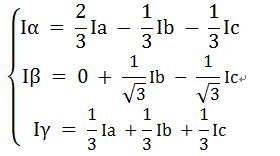将Ia+Ib+Ic=0代入上面的公式，可以得到：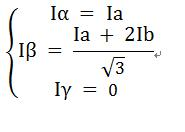我们需要关心的是Iα和Iβ，所以Clark变化最后的公式就是：

Iα = Ia ;

Iβ = (Ia + 2*Ib) / √3 ;

然后经过Park变换得到Iq, Id，他们是相互垂直的并且同时跟随着磁场方向在旋转，如下图：

如图七所示，Park变换是将静止的α，β电流变换成旋转的q轴和d轴电流，θ是旋转的角度，也称为电角度。

以电角度θ为夹角，分别对Iα,Iβ进行矢量分解，计算投影到q轴和d轴上的电流分量，可以得到以下公式：

Iq = Iβ*cosθ - Iα*sinθ

Id = Iα*cosθ + Iβ*sinθ

在计算得到Iq,Id之后，需要分别跟他们的设定值计算误差，然后分别做PI控制，得到Vq,Vd。

然后对Vq,Vd进行反Park变换，如下图：

在图八中，将Vq,Vd反向变换成Vα,Vβ，变换方式与Park变换类似，以电角度θ为夹角，分别对Vq,Vd进行矢量分解，计算投影到α轴和β轴上的电压分量，可以得到如下公式：

Vα = Vd*cosθ - Vq*sinθ;

Vβ = Vq*cosθ + Vd*sinθ;

在得到Vα和Vβ之后，需要通过SVPWM算法计算Va,Vb,Vc，关于SVPWM算法，网上有篇文章讲解的非常好，叫做《SVPWM的原理及法则推导和控制算法详解》，详细的内容可以去看这篇文章，下面就根据这篇文章做一些总结性的讲解。

SVPWM的全称是空间矢量脉宽调制(Space Vector Pulse Width Modulation)，是由三相功率逆变器的六个功率开关元件组成的特定开关模式产生的脉宽调制波，能够使输出电流波形尽可能接近于理想的正弦波形。理论基础是平均值等效原理，即在一个开关周期内通过对基本电压矢量加 以组合，使其平均值与给定电压矢量相等。

假设直流母线电压为Udc，三相相电压分别为UA，UB，UC，且相互之间的相位差为120°；假设Um为相电压有效值，f为电源频率，则有：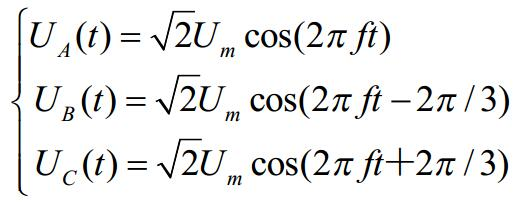则三相电压空间矢量相加的合成空间矢量U(t)就可以表示为：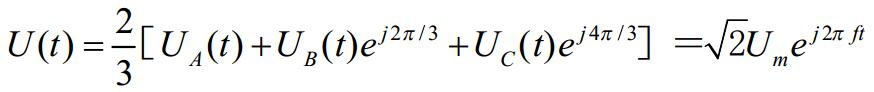U(t)是一个旋转的空间矢量，幅值不变，为相电压峰值，且以角频率ω=2πf按逆时针方向均匀旋转，而空间矢量U(t)在三相坐标轴(a,b,c)上的投影就是对称的三相正弦量。

下面讨论一下电压空间矢量：

图九是一个三相逆变电路，每一相在同一时刻只有一个桥会导通，定义这样一个开关函数Sx(x=a、b、c)：

上桥臂导通时，Sx=1；下桥臂导通时，Sx=0。举个例子：假设a相上桥导通，b和c相下桥导通，那么三相的结果就是a=1,b=0,c=0，组合的结果就是U4(100)。

在同一时刻，如果不同相的上下桥同时存在导通的桥，那么就会有相电流产生，属于非零矢量；如果同一时刻，三相的上桥同时导通或者三相的下桥同时导通，此时并不会有相电流产生，属于零矢量。所以总共存在6个非零矢量：U1(001)、U2(010)、U3(011)、U4(100)、U5(101)、U6(110)；以及两个零矢量：U0(000)、U7(111)。电压矢量的坐标如下图所示：

在图十中，显示了8个电压空间矢量U0~U7，以及六个扇区Ⅰ~Ⅵ。

假如Sx(x=a,b,c)=(100)，则此时Ua=⅔Udc，Ub=-⅓Udc，Uc=-⅓Udc，同理可以得到其他各种组合下的空间电压矢量，如下表：

结合图十和图十一可知，非零矢量的幅值相同，均为⅔Udc，相邻的矢量间隔60°，而两个零矢量幅值为0，位于中心。在每一个扇区，选择相邻两个电压矢量以及零矢量，按照伏秒平衡原则来合成每个扇区内的任意电压矢量，即：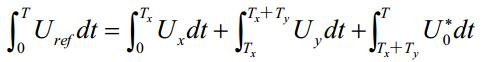或者等效成下式：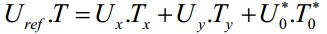其中，Uref为期望电压矢量，T为周期，Tx、Ty、T0*分别对应两个非零矢量Ux、Uy和零矢量U0*在一个周期T内的作用时间，其中U0*包括U0和U7两个零矢量。由于最终要得到的是作用在三相半桥上的占空比，也就是三个定时器通道的捕获比较寄存器的值，所以我们只要能计算出Tx、Ty、T0*的值，就可以知道三个捕获比较寄存器值。

先来看一下当Uref在第Ⅰ个扇区时的情况，见下图：

如图十二所示，Uref位于U4和U6之间，由正弦定理可得：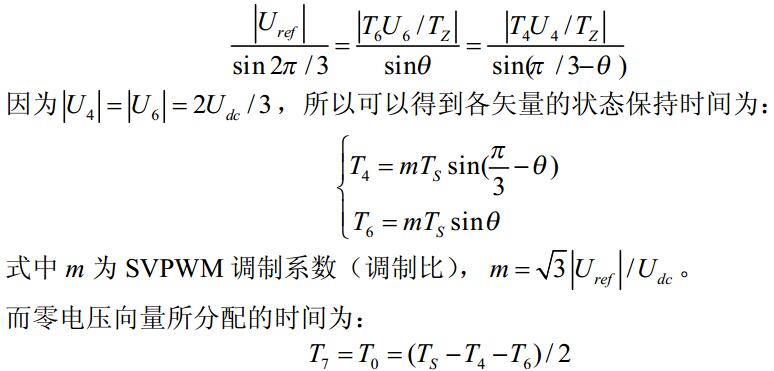得到以U4、U6、U7及U0合成的Uref的时间后，接下来就是如何产生实际的脉宽调制波形。在SVPWM调制方案中，零矢量的选择是最具灵活性的适当选择零矢量，可最大限度的减少开关次数，尽可能避免在负载电流较大的时刻的开关动作，最大限度的减少开关损耗。因此，我们以减少开关次数为目标，将基本矢量作用顺序的分配原则选定为：在每次开关状态转换时，只改变其中一相的开关状态，并且对零矢量在时间上进行了平均分配，以使产生的PWM对称，从而有效的降低PWM的谐波分量。可以发现当U4(100)切换至U0(000)时，只需改变A相上下一对切换开关，若由U4(100)切换至U7(111)则需要改变B、C相上下两对切换开关，增加了一杯的切换损失。因此要改变电压矢量U4(100)、U2(010)、U1(001)的大小，需配合零电压矢量U0(000)，而要改变U6(110)、U3(011)、U5(101)，需要配合零电压矢量U7(111)。这样通过在不同扇区内安排不同的开关切换顺序，就可以获得对称的输出波形，其他各扇区的开关切换顺序下表：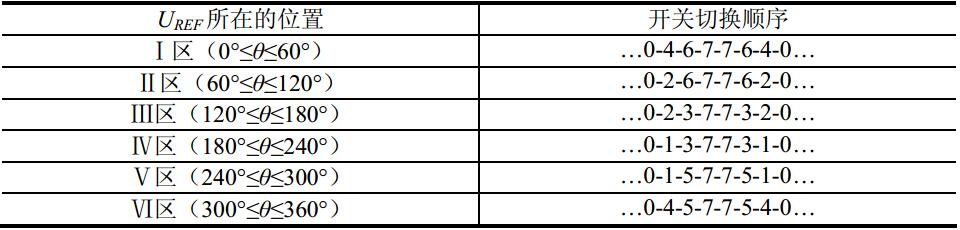同样以第Ⅰ扇区为例，电压矢量的先后顺序为U0、U4、U6、U7、U7、U6、U4、U0，将其画成a,b,c三相的PWM波形的话就如图十三所示：

一般我们在控制PMSM的时候都会将PWM波形设定为中央对齐模式，所以在图十三中，对称中心的两边各个电压矢量所占的时间都是该电压矢量在整个周期中所占时间的一半。

要先实现SVPWM的实时调制，我们首先要知道Uref所在的扇区位置，然后才能利用所在扇区的相邻电压矢量和适当的零矢量来合成电压矢量。由图十二可以知道，电压矢量Uref与α轴的夹角θ决定了Uref所在的扇区，所以我们只要判断θ角的大小即可知道Uref所在的扇区。

由 tanθ=Uβ/Uα，可知 θ=arctan(Uβ/Uα)，当Uref位于第Ⅰ扇区时，θ满足0<θ<60°，此时，Uα>0，Uβ>0，tanθ满足 0<tanθ<√3；所以Uref位于第Ⅰ扇区时的充要条件是：Uα>0，Uβ>0，√3Uα>Uβ。

同理可以得出Uref位于其他扇区时的等价条件，如下：

Uref位于第Ⅱ扇区时的充要条件是：Uβ>0，√3|Uα|<Uβ；

Uref位于第Ⅲ扇区时的充要条件是：Uα<0，Uβ>0，-√3Uα>Uβ；

Uref位于第Ⅳ扇区时的充要条件是：Uα<0，Uβ<0，√3Uα<Uβ；

Uref位于第Ⅴ扇区时的充要条件是：Uβ<0，√3|Uα|<-Uβ；

Uref位于第Ⅵ扇区时的充要条件是：Uα>0，Uβ<0，√3Uα>-Uβ；

进一步分析以上的条件就可以看出，电压矢量Uref所在的扇区完全由Uβ，√3Uα-Uβ，-√3Uα-Uβ三式决定，

因此令：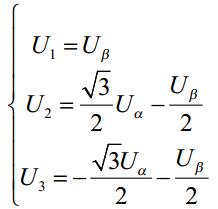再定义，若U1>0，则A=1，否则A=0；若U2>0，则B=1，否则B=0；若U3>0，则C=1，否则C=0。

再令N=4*C+2*B+A，则可以通过下标计算Uref所在的扇区。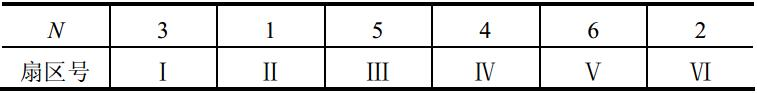接下来就要来计算合成Uref的相邻电压矢量的作用时间以及零矢量的作用时间。

假设Uref位于第Ⅰ扇区时(参照图十二)，用到的电压矢量是U4和U6，所以就需要计算T4和T6，以及零电压矢量的T0和T7。计算过程如下：

Uα*Ts = |U4|*T4 + |U6|*T6*cos(π/3)；

Uβ*Ts = |U4|*T4*0 + |U6|*T6*cos(π/6)；

前面说过，|U4|=|U6|=⅔Udc，所以有：

Uα*Ts = ⅔Udc*T4 + ⅓Udc*T6； ①

Uβ*Ts = (Udc*T6) / √3； ②

解①，②组成的方程组，得：

T4 = (√3/Udc) * Ts * (√3Uα/2 - Uβ/2) = (√3/Udc) * Ts * U2；

T6 = (√3/Udc) * Ts * Uβ = (√3/Udc) * Ts * U1；

又因为Ts=T0+T4+T6+T7，并且T0和T7的给定是灵活的，可以令T0=T7，那么就有：

T0 = T7 = (Ts - T4 - T6) / 2；

这样就计算出了Uref位于第Ⅰ扇区时各电压矢量的时间了。同理可以计算出位于其他扇区时的各电压矢量时间：

Uref位于第Ⅱ扇区时：

T2 = (-√3/Udc) * Ts * (√3Uα/2 - Uβ/2) = -(√3/Udc) * Ts * U2；

T6 = (-√3/Udc) * Ts * (-√3Uα/2 - Uβ/2) = -(√3/Udc) * Ts * U3；

T0 = T7 = (Ts - T2 - T6) / 2；

Uref位于第Ⅲ扇区时：

T2 = (√3/Udc) * Ts * Uβ = (√3/Udc) * Ts * U1；

T3 = (√3/Udc) * Ts * (-√3Uα/2 - Uβ/2) = (√3/Udc) * Ts * U3；

T0 = T7 = (Ts - T2 - T3) / 2；

Uref位于第Ⅳ扇区时：

T1 = (-√3/Udc) * Ts * Uβ = -(√3/Udc) * Ts * U1；

T3 = (-√3/Udc) * Ts * (√3Uα/2 - Uβ/2) = -(√3/Udc) * Ts * U2；

T0 = T7 = (Ts - T1 - T3) / 2；

Uref位于第Ⅴ扇区时：

T1 = (√3/Udc) * Ts * (-√3Uα/2 - Uβ/2) = (√3/Udc) * Ts * U3；

T5 = (√3/Udc) * Ts * (√3Uα/2 - Uβ/2) = (√3/Udc) * Ts * U2；

T0 = T7 = (Ts - T1 - T5) / 2；

Uref位于第Ⅵ扇区时：

T4 = (-√3/Udc) * Ts * (-√3Uα/2 - Uβ/2) = -(√3/Udc) * Ts * U3；

T5 = (-√3/Udc) * Ts * Uβ = -(√3/Udc) * Ts * U1；

T0 = T7 = (Ts - T4 - T5) / 2；

这样就完成了Uref位于6个扇区时的各电压矢量时间的计算，其中√3/Udc是SVPWM调制模式下的最大不失真电压幅值，在计算定时器各通道的捕获比较寄存器值时可以将√3/Udc直接去掉；Ts是PWM一个完整周期的时间，也就是定时器的计数周期。

在图十三中，整个PWM周期中，最先发生的是T0，接下来依次是T4，T6，T7，其中T4，T6是两个非零矢量，且T4先于T6发生，所以我们可以令先发生的非零矢量时间为Tx，后发生的非零矢量时间为Ty，并且T0=T7，那么就可以得出加载到三个捕获比较寄存器上的时间分别是：

T1 = (Ts - Tx - Ty) / 4；

T2 = T1 + Tx/2；

T3 = T2 + Ty/2；

只要根据Uref实际所在的扇区，确定Tx和Ty实际所对应的电压矢量，就可以计算出T1，T2，T3的值；然后再根据Uref所在的扇区画出类似图十三的三相PWM波形，就可以确定T1，T2，T3分别对应到三相A，B，C的哪一个通道，再赋值给对应通道的捕获比较寄存器，就完成了SVPWM算法。

至此，就完成了FOC控制，有哪里讲的不对的地方，希望可以指出。

展开全文SVPWM Clark
• 永磁同步电动机结构简单、体积小、重量轻、损耗小、效率高，和直流电机相比，它没有直流电机的换向器和电刷等缺点。和其他类型交流电动机相比，它由于没有励磁电流，因而效率高，功率因数高，力矩惯量比较大，定子...
• 便于读者对永磁同步电机型式实验项目进行学习，有利于对永磁类电机的技术开发
• 永磁同步电机 总体结构 转子磁路结构 气隙磁场 空载反电动势
• 永磁同步电机失磁故障分析，王刚，马宏忠，永磁同步电机具有高效率、高能量密度、结构简单等特点，在众多工业领域得到广泛应用。永磁同步电机在运行时容易受到温度、电枢反
• 由于是初学，对于simulink很多地方不熟悉，在... 永磁同步电机的英文缩写为PMSM，全称 Permanent Magnet Synchronous Machine。在 library 内搜索 Permanent 即可找到它。 Number of phase 电机相数 Back ...simulink 矢量控制
• 永磁同步电机的转子包括永磁体、转子铁芯、转轴、轴承等。 具体来说，根据永磁体在转子铁芯中的位置可以分为表面式和内置式PMSM。 其中表面式PMSM转子结构又分为：表贴式和插入式。 内置式PMSM转子磁路结构分为：...
• 1、永磁同步电机简介 三相永磁同步电机(Permanent Magnet Synchronous Motor, PMSM)按照转子结构的不同可分为: 表贴式永磁同步电机（Surface-mounted Permanent Magnet Synchronous Motor, SPMSM) 内置式永磁同步...数学建模 自动驾驶
• 导师研究的课题是永磁同步电机的控制，首先给我安排的任务就是将其矢量控制系统仿真搭建出来。本文记录矢量控制系统学习过程。因为是初学我的理解可能不够，其中每个内容的出处都会在文章内标注出来，大家可以参考...矢量控制 数学模型
• 3.1从PMSM电机的数学模型出发。 dq 轴 电压方程： dq 轴 轴磁链方程： dq 轴 转矩方程： dq 轴 运动方程： 分析上述方程，如果我们能够控制 id=0 那么电压方程就可简化为： ...PI调节器
• 1 永磁同步电机MTPA的控制原理 1.1 MTPA控制方式与id=0控制方式的区别 当电机采用id=0的控制策略，但是这种控制方法忽略和磁阻转矩的作用 。 这个从转矩方程最容易看出来，转矩分为永磁转矩Tr和磁阻转矩Tm，而id...控制原理
• ## 永磁同步电机

千次阅读 2020-10-11 17:08:18
永磁同步电机最大的优势在于其简单的结构，低廉的生产成本以及稳定的运行状态。本文的主要研究工作基于相坐标系，建立永磁同步发电机定子绕组匝间短路、转子磁场损失的数学模型，通过这些MATLAB程序，对系统进行仿真...
• 电机的分类 PMSM的机械组成 本书目的 三相PMSM的数学建模 三相PMSM的基本数学模型 PMSM转子结构分类 PMSM模型假设 PMSM的基本数学模型4个方程自然坐标系下 三相PMSM的坐标变换 Clark变换 iClark变换 Park...
• 1 弱磁扩速理论 PMSM弱磁的思想来源于他励直流电动机的调磁... 永磁同步电机的励磁磁通是由永磁体提供的，这个磁通是恒定不变的。这个时候如果我们想降低磁通强度，就只能通过增大定子电流的去磁分量来削弱气隙...PMSM 弱磁控制
• 1.0电机的分类及永磁同步电机的控制技术介绍 基础的电磁感应产生力的作用，这类似的初中物理知识不再讨论； 与直流无刷及永磁同步电机知识太发散的就不再讨论； 本文介绍的几点内容 电机的分类及日常生活...电机分类 无刷直流电机
• 1：此为永磁同步控制系列文章之一，应大家的要求，关于永磁同步矢量控制的系列文章已经在主页置顶，大家可以直接去主页里面查阅，希望能给大家带来帮助，谢谢。 2：矢量控制的六篇文章后。弱磁、MTPA、位置控制系列...矢量控制...